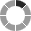Loading..Please Wait!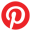Prepare forOlympiad Redeem Vouchers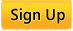Math Skills
 Practice Skills: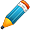Time Bound Skills: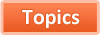Algebra
 Calendar
 Comparison
 Comparison And Estimation
 Counting
 Data Handling
 Decimals
 Division
 Estimation
 Exponents
 Factors
 Fractions
 Geometry
 Graphs
 Integers
 Location Vocabulary
 Measurement
 Mixed Operations
 Money
 Multiplication
 Number Sense
 Ordering
 Patterns
 Percentages
 Place Value
 Placement Assessments
 Probability
 Ratios And Proportions
 Roman Numerals
 Roots
 Rounding
 Shapes
 Standardized Mock Tests
 Statistics
 Subtraction
 Time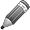I.01: Addition and subtraction up to 99 - Assessment 1I.02: Addition and subtraction up to 99 - Assessment 2I.03: Addition and subtraction up to 99 - Assessment 3I.04: Addition and subtraction up to 99 - Assessment 4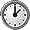I.05: Review on Addition and subtraction upto 99I.08: Story problems on addition and subtraction - Assessment 1I.09: Story problems on addition and subtraction - Assessment 2I.10: Review mixed addition and subtractionI.11: Balancing equations - addition and subtraction up to 10I.12: Balancing equations - addition and subtraction up to 20I.13: Balancing equations - addition and subtraction up to 30I.14: Balancing equations - addition and subtraction up to 40I.15: Balancing equations - addition and subtraction up to 50R.05: Review on number lineF.38.01: Complete the addition equations up to 3 digits - Assessment 1F.38.02: Complete the addition equations up to 3 digits - Assessment 2H.01: Mix of addition and subtraction for numbers up to three digits - Assessment 1H.02: Mix of addition and subtraction for numbers up to three digits - Assessment 2H.03: Mix of addition and subtraction for numbers up to three digits - Assessment 3H.04: Mix of addition and subtraction for numbers up to three digits - Assessment 4H.05: Review on addition and subtraction for numbers up to three digitsH.06: Which sign makes the addition or subtraction answer true?H.07.01: Find the missing number to make the answer true - Assessment 1H.07.02: Find the missing number to make the answer true - Assessment 2H.07.03: Review on finding the missing number to make the answer trueH.07.04: Find the missing number - Assessment 1H.07.05: Find the missing number - Assessment 2H.08: Story problems for 3 digit addition and subtraction - Assessment 1H.09: Story problems for 3 digit addition and subtraction - Assessment 2H.10: Review mixed addition and subtraction problemsH.11: Story problems that mix 2, 3 digit addition and subtraction in the same story - Assessment 1H.12: Story problems that mix 2, 3 digit addition and subtraction in the same story - Assessment 2C.18: Addition of numbers with 4 or more digits - Assessment 2E.01: Mixed review of addition and subtraction up to 4 digits - Assessment 1E.02: Mixed review of addition and subtraction up to 4 digits - Assessment 2E.03: Mixed review of addition and subtraction up to 4 digits - Assessment 3E.04.01: Mixed review of addition and subtraction up to 4 digits - Assessment 4E.04.02: Review on mixed addition and subtraction up to 4 digitsE.05: Story problems for addition and subtraction of numbers up to 4 digits - Assessment 1E.06: Story problems for addition and subtraction of numbers up to 4 digits - Assessment 2E.07: Addition and subtraction using parenthesis for up to 3 digitsE.08: Complete the equation for numbers up to 4 digitE.09: Addition and subtraction equations for numbers up to 4 digitsE.10: Review on mixed addition and subtraction in the same equationE.11: Story problems on multi step operations - Assessment 1E.12: Story problems on multi step operations - Assessment 2E.13: Mixed addition and subtraction for numbers with 4 or more digits - Assessment 1E.14: Mixed addition and subtraction for numbers with 4 or more digits - Assessment 2E.15: Review mixed addition and subtraction for numbers with 4 or more digitsE.16: Addition and subtraction equations for numbers with 4 or more digitsE.17: Story problems for addition and subtraction of numbers up to 6 digitsE.19: Which sign makes the equation trueE.20: Find the missing number in the equationE.21: Review addition and subtraction equationsD.01: Mixed addition and subtraction for numbers with 6 or more digits - Assessment 1D.02: Mixed addition and subtraction for numbers with 6 or more digits - Assessment 2D.06: Which sign makes the equation true - Assessment 1D.07: Which sign makes the equation true - Assessment 2D.08: Find the missing number in equation for numbers up to millions - Assessment 1D.09: Find the missing number in equation for numbers up to million - Assessment 2

Total Skills: 60

 Connect and Follow About Intelliseeds Learning My Account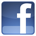Facebook Benefits Membership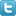Twitter Plans and Pricing Skills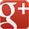Google+ FAQ Wall of Fame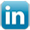LinkedIn Newsletter Student ReportsPinterest Careers Rewards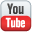Youtube Contact Us What is IntelliAbility?Our Partners Terms & Conditions Tell a FriendNon Profit Partners Testimonials User Guide PTA Fundraising Offers Common Core Math Schools Common Core ELA Virginia SOL Content Team
 Welcome to Intelliseeds Learning Smart learning for your child Intelliseeds learning provides practice in several subjects including Math, Reading Comprehension, Language Arts, Logical Reasoning, Mental Ability, Analytical Ability and Critical Thinking, which are essential to the success of every student. Skills are organized by grades, topics, practice tests and time bound tests. The Math section covers skills such as counting, number operations, algebra, story problems and many more. The IntelliAbility section focuses on thinking skills, patterns, sequences, problem analysis, analogies and over 50 other topics to help develop your child's brain. Click here to learn more about IntelliAbility. The Language Arts section includes reading comprehension, nouns, verbs, adverbs, adjectives, predicates, vowel sounds and many more grammar skills.Privacy | © 2020 Intelliseeds Learning™